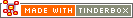* (multiplication)Operator Type: Mathematical Operator Scope of Action: Item

The multiplication operator, * (asterisk character), returns the multiplication of the arguments before and after it.

`\$MyNumber = 3*4`

The multiplication operator * may be used to multiply strings. Thus, the expression

`\$MyString = 3*"xyz"`

evaluates to a string "xyzxyzxyz"

 Up: Item-based operators Previous: &= (logical AND) Next: + (addition)

[Last updated: 14 Dec 2009, using v5.0]